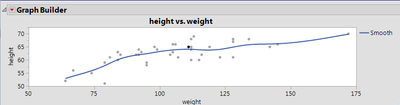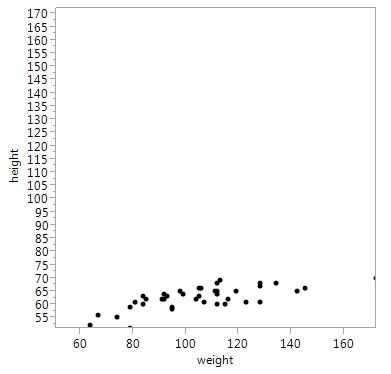Choose Language Hide Translation Bar
Highlighted

## Setting both axis to the same range

The default range setting in Y by X graphs is to show the full range of the data independently in x and y.  This is fine, but I have a special condition where I an plotting some residual errors in the x and y direction and I would like the graphs to have the same range in both x and y.  The effect I am trying to achieve is that if the errors are normally distributed in each direction then the residuals so show up in a circular pattern. If they are worse in either X or Y a ellipse should be seen.  Of course there are outliers in each direction and the axis range is being set by them.

What is the easiest way to script the axis to take the full range of the worse direction?  Something akin to uniform scaling in histogram plots.

1 ACCEPTED SOLUTION

Accepted Solutions
Highlighted

## Re: Setting both axis to the same range

interactively, right-click-graph->Graph->Size/Scale->Size To Isometric.

`g = Graph Builder(  Size( 348, 336 ),  Show Control Panel( 0 ),  Variables( X( :weight ), Y( :height ) ),  Elements( Points( X, Y, Legend( 6 ) ), Smoother( X, Y, Legend( 7 ) ) ));(report(g)[framebox(1)])<<size to isometric;`

the graph starts out about square, 348x336.  size to isometric:The two scales match, 50 to 70 on the v-axis is a little shorter than 50 to 75 on the h-axis.

Craige
4 REPLIES 4
Highlighted

## Re: Setting both axis to the same range

interactively, right-click-graph->Graph->Size/Scale->Size To Isometric.

`g = Graph Builder(  Size( 348, 336 ),  Show Control Panel( 0 ),  Variables( X( :weight ), Y( :height ) ),  Elements( Points( X, Y, Legend( 6 ) ), Smoother( X, Y, Legend( 7 ) ) ));(report(g)[framebox(1)])<<size to isometric;`

the graph starts out about square, 348x336.  size to isometric:The two scales match, 50 to 70 on the v-axis is a little shorter than 50 to 75 on the h-axis.

Craige
Highlighted
Highlighted

## Re: Setting both axis to the same range

Craige's answer is probably the easiest. We often need a square version of the graph with the axes spanning the entire range. One way to do that is to determine the min and max values and set both axes to the same values.

g = Bivariate( Y( :height ), X( :weight ) );

minaxis = Min( Col Min( :height ), Col Min( :weight ) );

maxaxis = Max( Col Max( :height ), Col Max( :weight ) );

(Report( g )[axisbox( 1 )]) << {Min( minaxis ), Max( maxaxis )};

(Report( g )[axisbox( 2 )]) << {Min( minaxis ), Max( maxaxis )};

(Report( g )[framebox( 1 )]) << Frame Size( 320, 320 );

The resulting graph:I sometimes add a 45-degree line to this graph to indicate X=Y. This graph is useful if X and Y represent the same property or characteristic (not height and weight). If X and Y represented values of the same characteristic measured by measurement devices X and Y, this graph would tell us that there is a problem between the two measurement devices.

Some points are on the edge of the graph. This can be fixed by using a "weighted combination" of min and max values to widen the axes:

minaxis = 1.05 * Min( Col Min( :height ), Col Min( :weight ) ) - 0.05 * Max( Col Max( :height ), Col Max( :weight ) );

maxaxis = -0.05 * Min( Col Min( :height ), Col Min( :weight ) ) + 1.05 * Max( Col Max( :height ), Col Max( :weight ) );

Good luck!

Howard

Highlighted

## Re: Setting both axis to the same range

Hi Howard,

Your solution is the more desired.  I would suggest that this be a feature request to be settable like isometric.

Andy

Article Labels

There are no labels assigned to this post.